• 精品文档 精品文档 PAGE PAGE #欢迎下载 实验六拉普拉斯变换及其逆变换 目的 掌握连续系统及信号拉普拉斯变换概念 掌握利用MATLAB^制系统零极点图的方法 掌握利用MATLAB^解拉普拉斯逆变换的方法 二 拉普拉斯变换...
• 但是，一般的教材上来就是拉普拉斯变换的数学定义，对于其历史和代表的深刻含义没有任何介绍，导致很多人一直头疼不已。今天，我们尝试一下从不同的角度来看看拉普拉斯变换到底是怎么回事？先从个人说起：奥列弗...
一、奥列弗. 赫维赛德是何许人也
二、傅里叶变换（轻量版拉普拉斯变换）
三、拉普拉斯变换（原来就是那么回事）

拉普拉斯变换可以说是现代工程学使用最广泛的数学工具，它通过数学变换将微积分方程转化成代数方程，为求解连续空间连续时间的方程提供了可能。但是，一般的教材一上来就是拉普拉斯变换的数学定义，对于其历史和代表的深刻含义没有任何介绍，导致很多人一直头疼不已。今天，我们尝试一下从不同的角度来看看拉普拉斯变换到底是怎么回事？先从一个人说起：奥列弗.赫维赛德，一位在科学史地位被严重低估的人。

一、奥列弗. 赫维赛德是何许人也

奥列弗. 赫维赛德（Oliver Heaviside）是维多利亚时期英国人，出身于极度贫穷的家庭，听力部分残疾，还得过猩红热，从未上过大学，完全靠自学和兴趣掌握了高等科学和数学。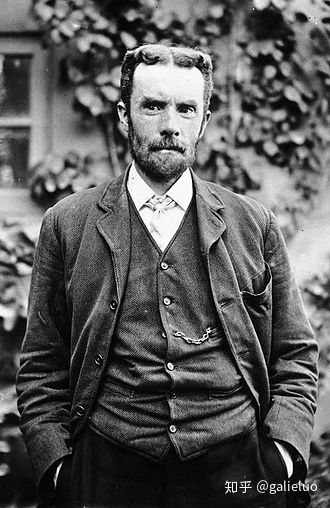很多人熟悉赫维赛德是因为MATLAB有一个赫维赛德（Heaviside）函数，它大概长这个样子，可以看成一个阶跃函数，这个函数因为和狄拉克（Dirac）函数之间的千丝万缕的关系而显得尤为重要。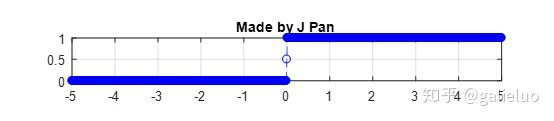我们现在说赫维赛德，当然不是因为这个函数，而是因为我们叹为观止、惊为天人，怎么夸都不过分的麦克斯韦方程组，麦克斯韦本人并没有见过这个方程组，它在一定程度上应该叫“赫维赛德方程组”。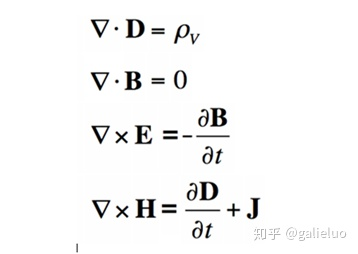这四个公式简直太对称了！而且它们的含义也很清晰：变化的电场产生磁场，变化的磁场产生电场，电磁波也就是电场和磁场此消彼长、相互转化、向前传播的形式。多亏了赫维赛德，麦克斯韦的理论才得以在十九世纪结束之前就真正站稳了脚跟并发扬光大。可以毫不谦虚的说：宇宙间任何的电磁现象，皆可由麦克斯韦方程组解释，包括光。也正是因为这个方程组完美统一了整个电磁场，让爱因斯坦始终想要以同样的方式统一引力场，并将宏观与微观的两种力放在同一组式子中：即著名的“大一统理论”，不幸的是麦克斯韦成功了，而爱因斯坦没有。

麦克斯韦早在1873年便出版了跨时代巨著《电磁通论》，可惜的是，他英年早逝，他的方程组在生前并没有得到科学界的关注，其中一个很重要的原因是他的理论描述复杂得令人吃惊，他最初提出的电磁理论公式包含了二十个方程，直接导致了他的理论在首次发表后的10多年时间内，几乎无人问津。

赫维赛德最伟大的贡献是简化了麦克斯韦的原始方程组，通过他天才般的洞察力，挖掘出了蕴含在麦克斯韦方程内部的深刻意义，从而使简化后麦克斯韦方程组呈现出无与伦比的对称性，成为历史上是最漂亮的方程式（没有唯一）。

而我们今天要说的，是赫维赛德的第二个重要贡献：运算微积分。学过电磁学的人都知道，在历史上人们发现好多定理公式，都是用微积分的形式表达的。1880年-1887年之间，赫维赛德在从事电磁场研究的同时，为求解微积分方程，在他的分析计算中引入了微分算子的概念，这个方法牛逼在什么地方呢？——它可以将常微分方程转换为普通代数方程。天才与普通人的区别就是人家是靠“直觉”来解决问题的。赫维赛德是怎么解微分方程的呢？他把微分、积分运算用一个简单的算子来代替。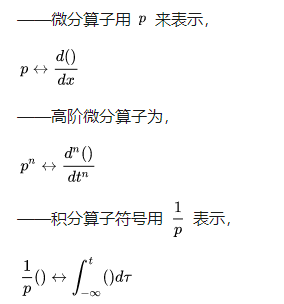也就是说，在某种算子下，积分和微分对应的是倒数关系，至于算子   代表什么，赫维赛德也没有多解释，在缺乏严密数学基础的情况下，人家直接放在文章就用了，还发表了。比如常见的一个二阶常微分方程，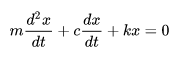用赫维赛德的微分算子变换一下，就变成了代数表达式，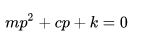赫维赛德之所以这么做，是因为他的“物理直觉”告诉他这么做，就是这么硬。这显然是一种开外挂的行为，因此也受到当时的主流数学家们们的攻讦，他们认为赫维赛德就是十足的“民科”，文章没什么理论依据，自己在那空想呢。当然，赫维赛德也不是弱鸡，科学家怼起人来，也是毫不含糊：“因为我不能理解消化过程就拒绝晚餐吗？不，只要我满意这个结果。”

好了，扯了那么远，有童鞋已经不耐心了：这些和拉普拉斯变换有什么关系？谜底就是：赫维赛德的微积分算子，就是拉普拉斯变换的前身。

赫维赛德的算子验算虽然缺乏严密的数学基础，往往能给出重要且正确的结果，方法确实有效，无法驳倒。于是在世纪之交，数学家们开始尝试随算子理论进行严格化。后来，人在在70年前法国数学家拉普拉斯的一本有关概率论的著作上，找到了这种算法的依据，但是这本书上提出的并不是现在我们看到的拉普拉斯变换，而是著名的Z变换。什么？拉普拉斯变换不是拉普拉斯提出的？随着二战后拉普拉斯变换的广泛使用，赫维赛德算子的作用被弱化了，但是不可否认的是，这是这种“不正规”，仅靠“天才的直觉”而发明的方法，促成了现在拉普拉斯分析法。

二、傅里叶变换（轻量版拉普拉斯变换）

在说拉普拉斯变换以前，我们要先提一下傅里叶变换，这可以看成是轻量版的拉普拉斯变换。傅里叶变换说的是什么事？说的是自然界的很多现象，都可以用三角函数进行分解。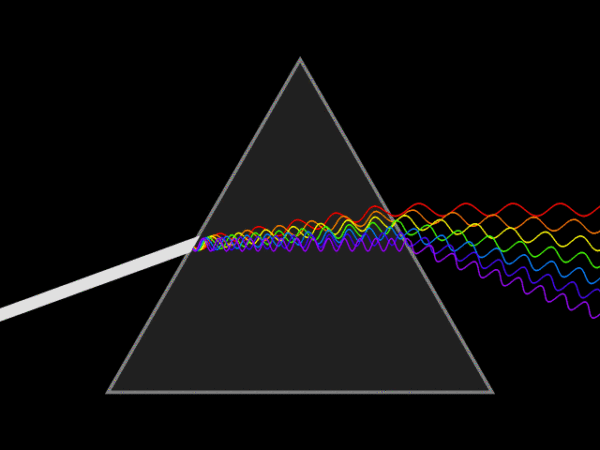古巴比伦科学家在很早就用了三角函数和逼近的方法，对天体运动进行观测和预报，1748年，大神欧拉用类似的方法分析可弦的振动（大神就是高产，哪哪都有他的身影），1753年伯努利提出任意物理弦的振动都可以可以表达为三角函数的和，但是他没给出证明（注意，伯努利是一个家族，3代人中产生了8位科学家，后裔有不少于120位被人们系统地追溯过）。1807年，傅里叶于年在法国科学学会上提交了一篇论文，运用正弦曲线来描述温度分布，论文里有个在当时具有争议性的观点：任何连续周期信号可以由一组适当的正弦曲线组合而成。傅里叶没有做出严格的数学论证。这篇论文的审稿人中，有历史上著名的数学家拉格朗日和拉普拉斯，当拉普拉斯和其它审稿人投票通过并要发表这篇论文时，拉格朗日坚决反对，认为傅里叶的方法无法表示带有棱角的信号。法国科学学会屈服于拉格朗日的威望，拒绝了傅里叶的工作。1822年，傅里叶变换随其著作《热的解析》发表，但已经是15年之后了。1829年，狄利赫里通过推导其适用范围，完善了傅里叶变换。

我们知道，三角函数可以通过欧拉公式与复指数函数联系起来，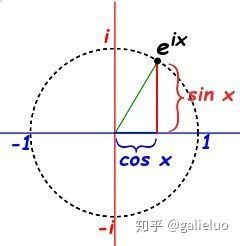也就是说，复指数函数是与正弦函数、余弦函数紧密相关的，那是不是某些信号也能通过复指数函数进行分解呢？我们不妨先来研究一下   有什么特殊的性质。

在《自然常数“e”，工程中的自然数“1”》、《傅里叶变换后面的到底有什么小秘密》、《被众人膜拜的欧拉恒等式是个什么东东？》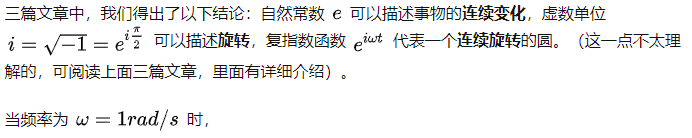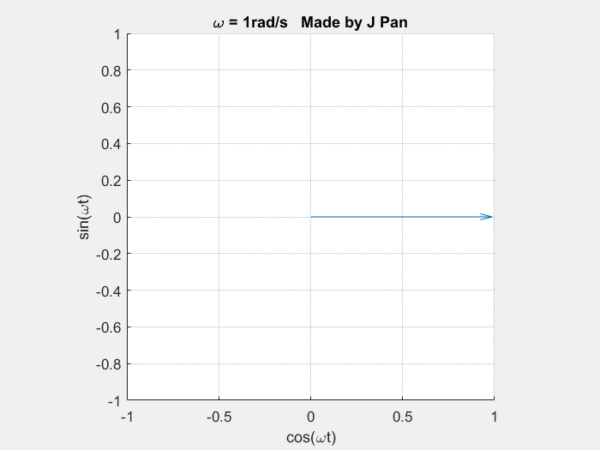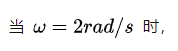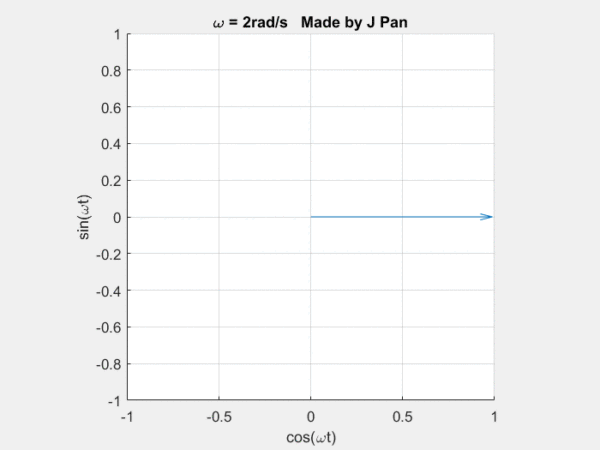clc;clear;
h = animatedline;
xl=xlabel('cos(\omegat)');%
yl=ylabel('sin(\omegat)');%
grid on;
axis([-1,1,-1,1]);
axis square;
N = 100;
t=linspace(0,2*pi,N);
w=1;
x=cos(w*t);
y=sin(w*t);
a = tic; % start timer
for k = 1:N
hold on
quiver(0,0,x(k)*1.1,y(k)*1.1)
b = toc(a); % check timer
if b > (1/90)
drawnow % update screen every 1/30 seconds
a = tic; % reset timer after updating
end
end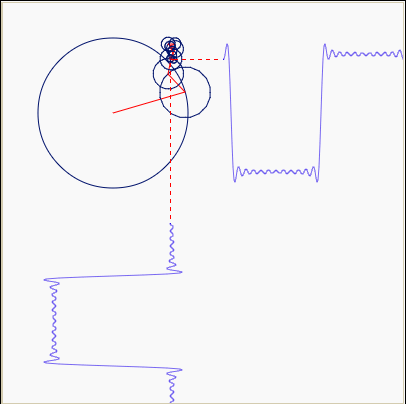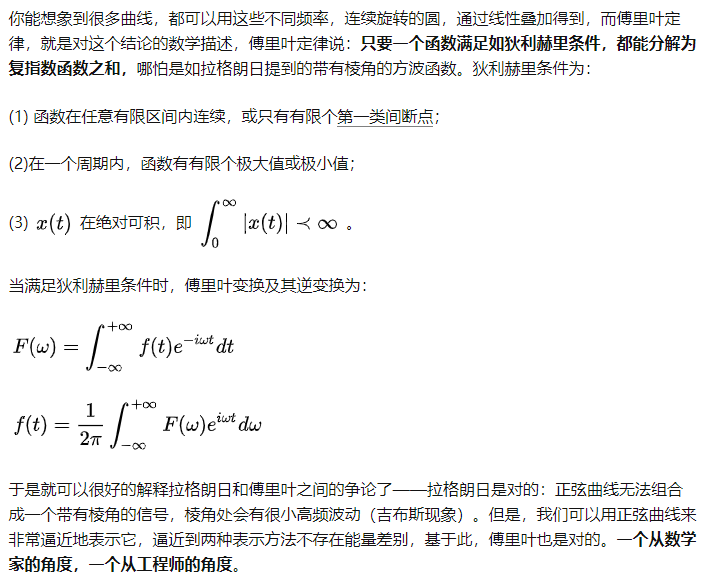三、拉普拉斯变换（原来就是那么回事）

傅里叶变换能帮我们解决很多问题，一经问世后便受到广大工程师们的喜爱，因为它给人们提供了一扇不同的窗户来观察世界，从这个窗户来看，很多事情往往变得简单多了。但是，别忘了，傅里叶变换有一个很大局限性，那就是信号必须满足狄利赫里条件才行，特别是那个绝对可积的条件，一下子就拦截掉了一大批函数。比如函数 f(t)=t^2 就无法进行傅里叶变换。这点难度当然拿不到聪明的数学家们，他们想到了一个绝佳的主意：把不满足绝对的可积的函数乘以一个快速衰减的函数，这样在趋于无穷时原函数也衰减到零了，从而满足绝对可积。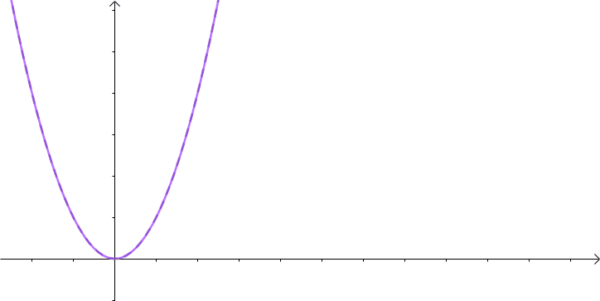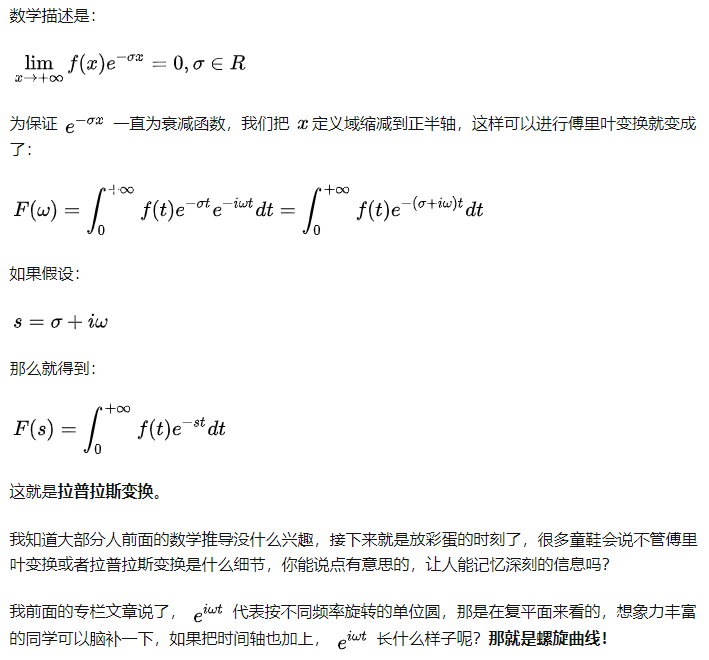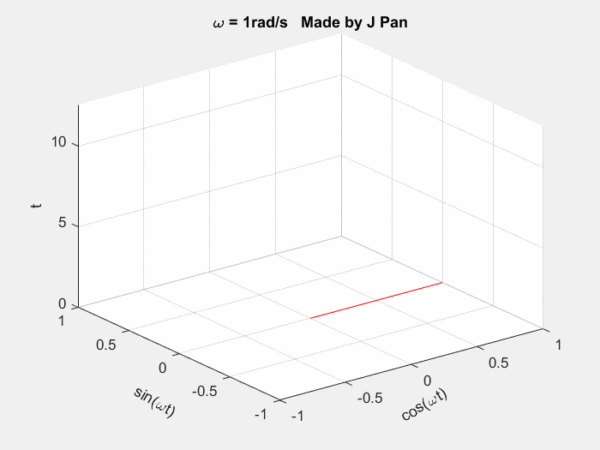clc;clear;
h = animatedline;
h1=gcf;
view(3);
xl=xlabel('cos(\omegat)');%
yl=ylabel('sin(\omegat)');%
zl=zlabel('t');%
set(xl,'Rotation',30);%
set(yl,'Rotation',-30);%
grid on;
axis([-1,1,-1,1,0,4*pi])
N = 200;
t=linspace(0,4*pi,N);
w=1;
x=cos(w*t);
y=sin(w*t);
a = tic; % start timer
for k = 1:N
hold on
line([0 x(k)],[0 y(k)],[t(k) t(k)],'Color','red')
b = toc(a); % check timer
if b > (1/90)
drawnow % update screen every 1/30 seconds
a = tic; % reset timer after updating
end
end

从其他不同的平面看，螺旋曲线就是正弦或者余弦曲线。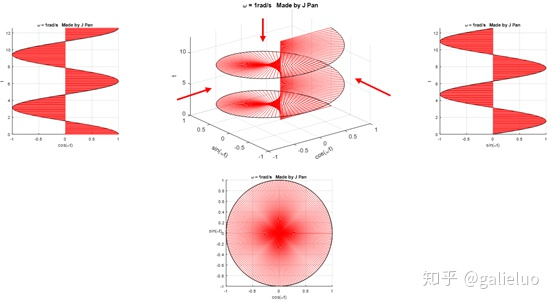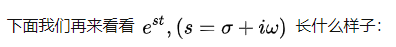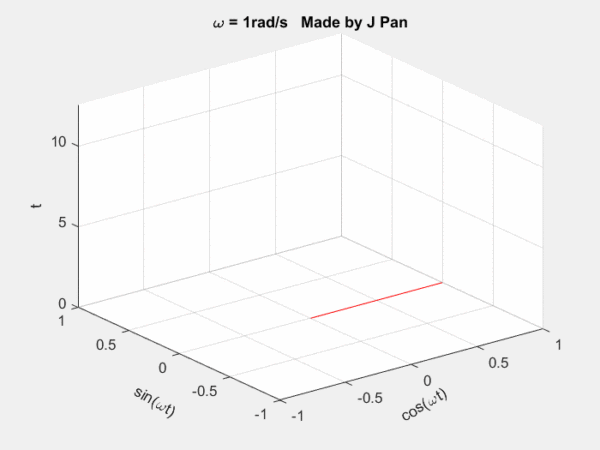clc;clear;
h = animatedline;
h1=gcf;
view(3);
xl=xlabel('cos(\omegat)');%
yl=ylabel('sin(\omegat)');%
zl=zlabel('t');%
set(xl,'Rotation',30);%
set(yl,'Rotation',-30);%
grid on;
axis([-1,1,-1,1,0,4*pi])
N = 200;
t=linspace(0,4*pi,N);
w=1;sig=-0.2;
x=exp(sig*t).*cos(w*t);
y=exp(sig*t).*sin(w*t);
a = tic; % start timer
for k = 1:N
hold on
line([0 x(k)],[0 y(k)],[t(k) t(k)],'Color','red')
b = toc(a); % check timer
if b > (1/90)
drawnow % update screen every 1/30 seconds
a = tic; % reset timer after updating
end
end

螺旋曲线和衰减函数的乘积：一个半径不断减小的螺旋曲线。从不同的平面看，就是不断衰减的正弦或者余弦曲线，从复平面来看，是一个半径不断减小的圆。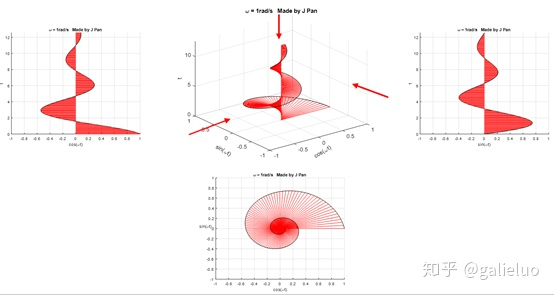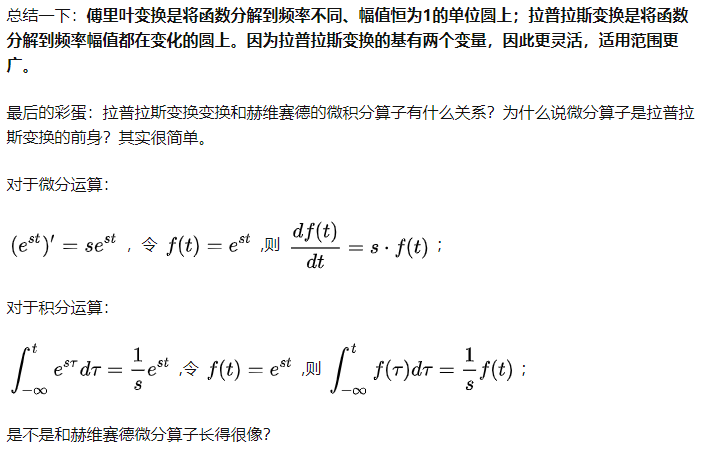展开全文数学建模 信号处理 信号与系统 傅里叶变换
• ## 拉普拉斯变换

万次阅读 多人点赞 2019-04-26 11:06:15
本文将从通俗的角度看待拉普拉斯变换。 发明者 奥列弗.赫维赛德，维多利亚时期英国人，全靠自学，听力残疾。很多人熟悉赫维赛德是因为MATLAB有个赫维赛德（Heaviside）函数。 赫维赛德简化了麦克斯韦方程组：即...


本文将从通俗的角度看待拉普拉斯变换。

发明者

奥列弗.赫维赛德，维多利亚时期英国人，全靠自学，听力残疾。很多人熟悉赫维赛德是因为MATLAB有一个赫维赛德（Heaviside）函数。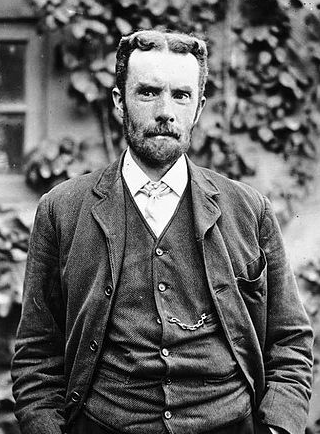赫维赛德简化了麦克斯韦方程组：即变化的电场产生磁场，变化的磁场产生电场。让20个方程组便成了4个。
**赫维赛德另一个贡献就是我们今天要说的运算微积分-它可以将常微分方程转换为普通代数方程。**赫维赛德是怎么解微分方程的呢？他把微分、积分运算用一个简单的算子来代替。也就是说，在某种算子下，积分和微分对应的是倒数关系，至于算子 p 代表什么，赫维赛德也没有多解释，在缺乏严密数学基础的情况下，人家直接放在文章就用了，还发表了。比如常见的一个二阶常微分方程，如果用赫维赛德的微分算子变换一下，就变成了代数表达式。赫维赛德之所以这么做，是因为他的“物理直觉”告诉他这么做，就是这么硬。这显然是一种开外挂的行为，因此也受到当时的主流数学家们们的攻讦，他们认为赫维赛德就是十足的“民科”，文章没什么理论依据，自己在那空想呢。当然，赫维赛德也不是弱鸡，科学家怼起人来，也是毫不含糊：“因为我不能理解消化过程就拒绝晚餐吗？不，只要我满意这个结果。”
好了，扯了那么远，有童鞋已经不耐心了：这些和拉普拉斯变换有什么关系？谜底就是：赫维赛德的微积分算子，就是拉普拉斯变换的前身。

傅里叶变换（轻量版拉普拉斯变换）

在说拉普拉斯变换以前，我们要先提一下傅里叶变换，这可以看成是轻量版的拉普拉斯变换。傅里叶变换说的是什么事？说的是自然界的很多现象，都可以用三角函数进行分解。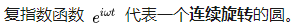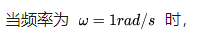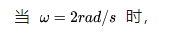clc;clear;
h = animatedline;
xl=xlabel('cos(\omegat)');%
yl=ylabel('sin(\omegat)');%
grid on;
axis([-1,1,-1,1]);
axis square;
N = 100;
t=linspace(0,2*pi,N);
w=1;
x=cos(w*t);
y=sin(w*t);
a = tic; % start timer
for k = 1:N
hold on
quiver(0,0,x(k)*1.1,y(k)*1.1)
b = toc(a); % check timer
if b > (1/90)
drawnow % update screen every 1/30 seconds
a = tic; % reset timer after updating
end
end
123456789101112131415161718192021222324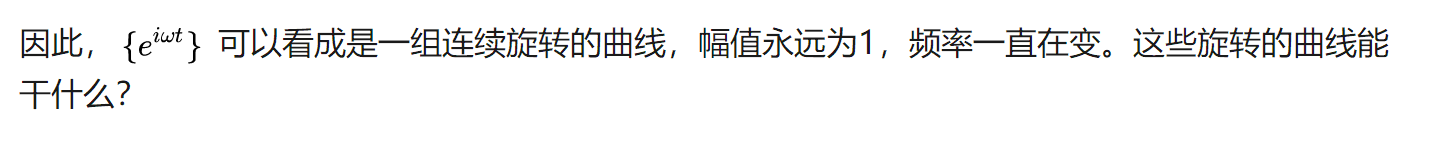你能想象到很多曲线，都可以用这些不同频率，连续旋转的圆，通过线性叠加得到，而傅里叶定律，就是对这个结论的数学描述。
傅里叶定律说：只要一个函数满足如狄利赫里条件，都能分解为复指数函数之和，哪怕是如拉格朗日提到的带有棱角的方波函数。狄利赫里条件为：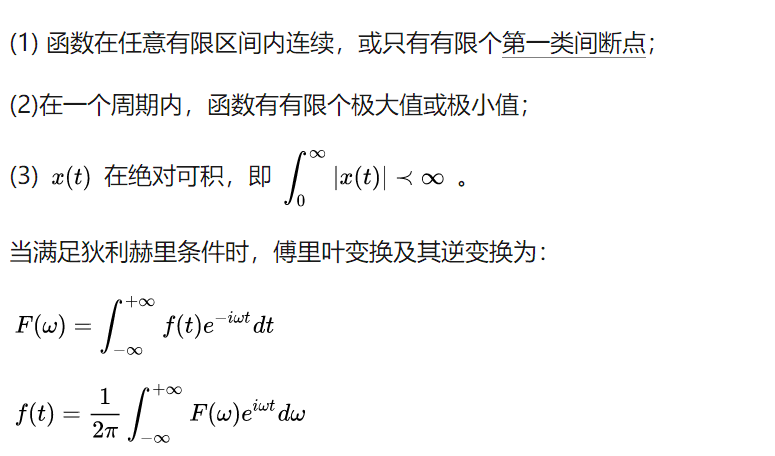其中可去间断点和跳跃间断点属于第一类间断点
于是就可以很好的解释拉格朗日和傅里叶之间的争论了——拉格朗日是对的：正弦曲线无法组合成一个带有棱角的信号，棱角处会有很小高频波动（吉布斯现象）。但是，我们可以用正弦曲线来非常逼近地表示它，逼近到两种表示方法不存在能量差别，基于此，傅里叶也是对的。一个从数学家的角度，一个从工程师的角度。

拉普拉斯变换-原来就是这么回事
傅里叶变换能帮我们解决很多问题，一经问世后便受到广大工程师们的喜爱，因为它给人们提供了一扇不同的窗户来观察世界，从这个窗户来看，很多事情往往变得简单多了。但是，别忘了，傅里叶变换有一个很大局限性，那就是信号必须满足狄利赫里条件才行，特别是那个绝对可积的条件，一下子就拦截掉了一大批函数。比如函数 f(t)=t^2 就无法进行傅里叶变换。这点难度当然拿不到聪明的数学家们，他们想到了一个绝佳的主意：把不满足绝对的可积的函数乘以一个快速衰减的函数，这样在趋于无穷 时原函数也衰减到零了，从而满足绝对可积。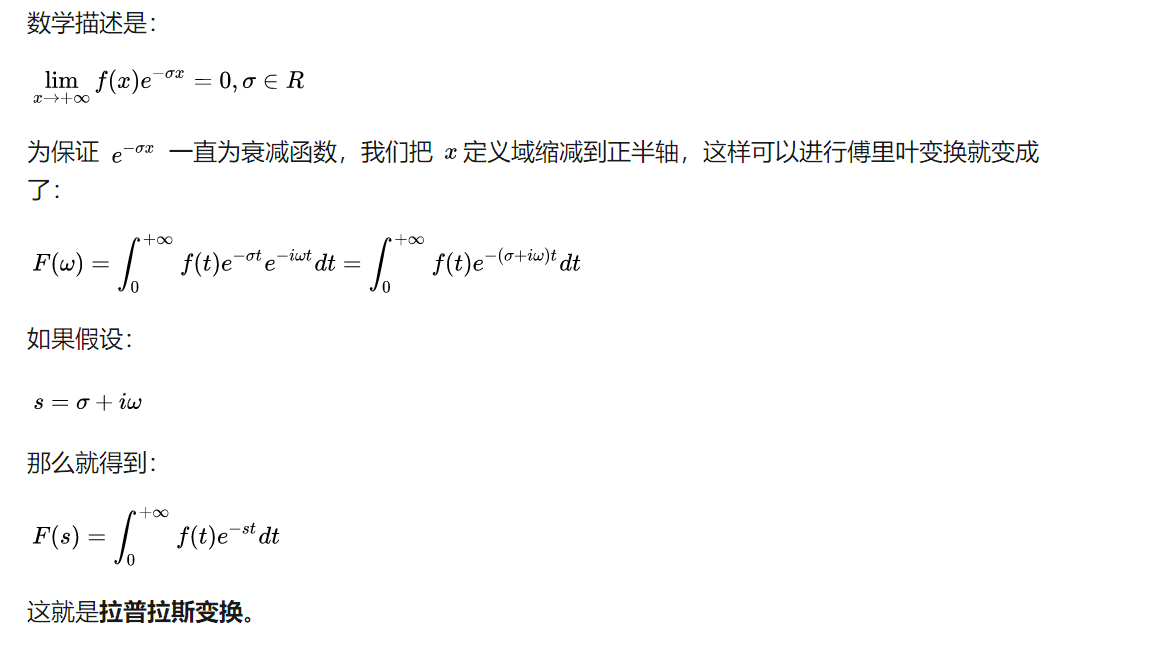这里我要补充一下，不是为了保证一直为衰减，指数函数，要衰减，在负半轴也是衰减的，要增加，在正负半轴都是增加的。是因为在我们关心的系统中，不对时间的负半轴作分析。因此，我们更多使用单边的拉普拉斯变换，而不是使用双边的拉普拉斯变换，这样的系统称之为因果系统不需要考虑 t=0 时的系统初始条件。
我知道大部分人前面的数学推导没什么兴趣，接下来就是放彩蛋的时刻了，很多童鞋会说不管傅里叶变换或者拉普拉斯变换是什么细节，你能说点有意思的，让人能记忆深刻的信息吗？clc;clear;
h = animatedline;
h1=gcf;
view(3);
xl=xlabel('cos(\omegat)');%
yl=ylabel('sin(\omegat)');%
zl=zlabel('t');%
set(xl,'Rotation',30);%
set(yl,'Rotation',-30);%
grid on;
axis([-1,1,-1,1,0,4*pi])
N = 200;
t=linspace(0,4*pi,N);
w=1;
x=cos(w*t);
y=sin(w*t);
a = tic; % start timer
for k = 1:N
hold on
line([0 x(k)],[0 y(k)],[t(k) t(k)],'Color','red')
b = toc(a); % check timer
if b > (1/90)
drawnow % update screen every 1/30 seconds
a = tic; % reset timer after updating
end
end
12345678910111213141516171819202122232425262728clc;clear;
h = animatedline;
h1=gcf;
view(3);
xl=xlabel('cos(\omegat)');%
yl=ylabel('sin(\omegat)');%
zl=zlabel('t');%
set(xl,'Rotation',30);%
set(yl,'Rotation',-30);%
grid on;
axis([-1,1,-1,1,0,4*pi])
N = 200;
t=linspace(0,4*pi,N);
w=1;sig=-0.2;
x=exp(sig*t).*cos(w*t);
y=exp(sig*t).*sin(w*t);
a = tic; % start timer
for k = 1:N
hold on
line([0 x(k)],[0 y(k)],[t(k) t(k)],'Color','red')
b = toc(a); % check timer
if b > (1/90)
drawnow % update screen every 1/30 seconds
a = tic; % reset timer after updating
end
end
12345678910111213141516171819202122232425262728
螺旋曲线和衰减函数的乘积：一个半径不断减小的螺旋曲线。从不同的平面看，就是不断衰减的正弦或者余弦曲线，从复平面来看，是一个半径不断减小的圆。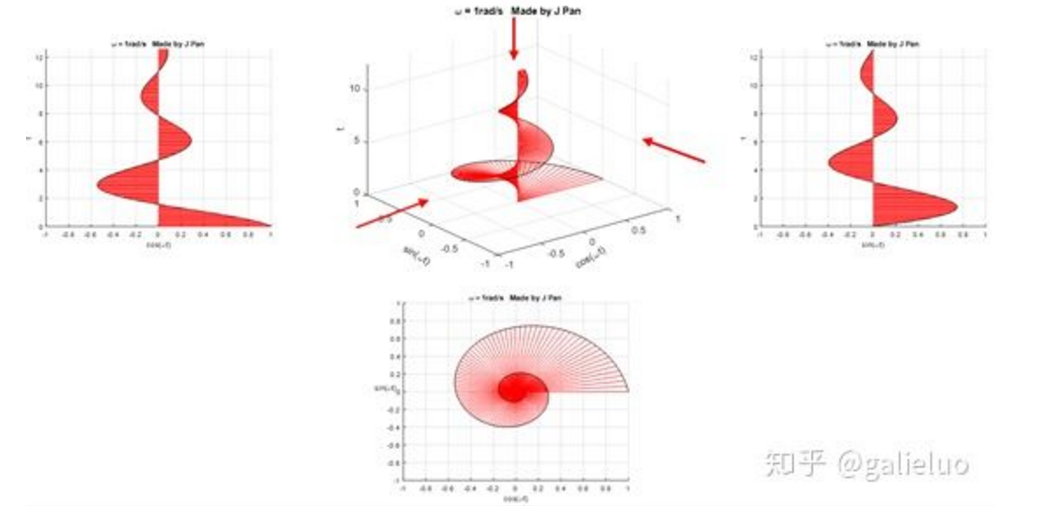https://zhuanlan.zhihu.com/p/40783304

总结一下：傅里叶变换是将函数分解到频率不同、幅值恒为1的单位圆上；拉普拉斯变换是将函数分解到频率幅值都在变化的圆上。因为拉普拉斯变换的基有两个变量，因此更灵活，适用范围更广。
本文大量引用了
https://zhuanlan.zhihu.com/p/40783304
对此表示感谢
        </div>
</div>



展开全文• 我们新的数值拉普拉斯逆变换过程快速，简单，但仍比最常用的替代过程优越得多。 它没有过冲和下冲，因此非常适合反转概率分布的拉普拉斯变换，因为它永远不会为它们返回负值或个以上的结果。matlab
• 第三节 连续信号的拉普拉斯变换...拉普拉斯变换与傅立叶变换的关系 由零极点图对傅立叶变换进行几何求解 * 一拉普拉斯变换 1从傅立叶变换到拉普拉斯变换 有几种情况不满足狄里赫利条件 指数增长信号 功率型周期信号 若
• 从另个角度看拉普拉斯变换 J Pan 航空工程师 、奥列弗. 赫维赛德是何许人也 二、傅里叶变换（轻量版拉普拉斯变换） 三、拉普拉斯变换（原来就是那么回事） 拉普拉斯变换可以说是现代工程学使用最广泛的数学工具...
全文转载自 https://zhuanlan.zhihu.com/p/40783304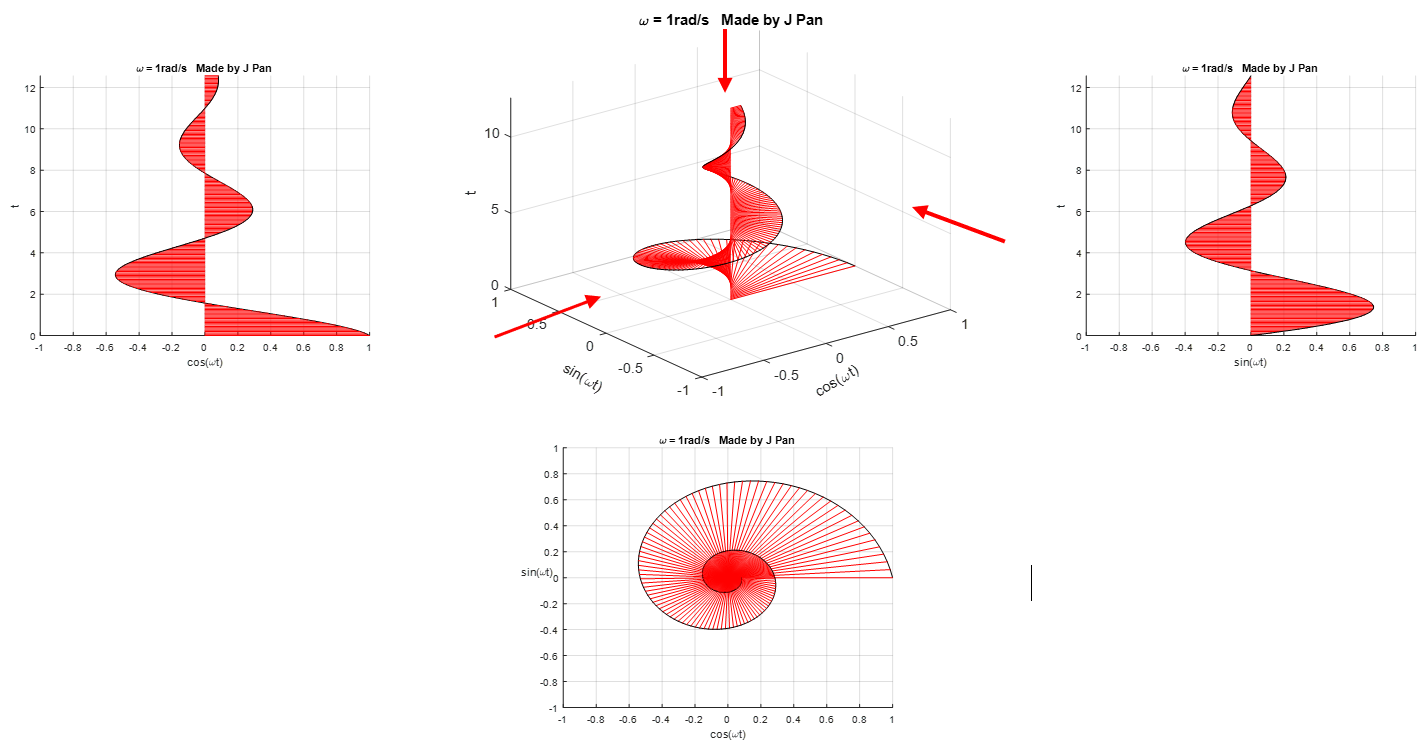从另一个角度看拉普拉斯变换
J Pan
航空工程师
一、奥列弗. 赫维赛德是何许人也
二、傅里叶变换（轻量版拉普拉斯变换）
三、拉普拉斯变换（原来就是那么回事）
拉普拉斯变换可以说是现代工程学使用最广泛的数学工具，它通过数学变换将微积分方程转化成代数方程，为求解连续空间连续时间的方程提供了可能。但是，一般的教材一上来就是拉普拉斯变换的数学定义，对于其历史和代表的深刻含义没有任何介绍，导致很多人一直头疼不已。今天，我们尝试一下从不同的角度来看看拉普拉斯变换到底是怎么回事？先从一个人说起：奥列弗.赫维赛德，一位在科学史地位被严重低估的人。

一、奥列弗. 赫维赛德是何许人也
奥列弗. 赫维赛德（Oliver Heaviside）是维多利亚时期英国人，出身于极度贫穷的家庭，听力部分残疾，还得过猩红热，从未上过大学，完全靠自学和兴趣掌握了高等科学和数学。图片来自：https://en.wikipedia.org/wiki/Oliver_Heaviside
很多人熟悉赫维赛德是因为MATLAB有一个赫维赛德（Heaviside）函数，它大概长这个样子，可以看成一个阶跃函数，这个函数因为和狄拉克（Dirac）函数之间的千丝万缕的关系而显得尤为重要。我们现在说赫维赛德，当然不是因为这个函数，而是因为我们叹为观止、惊为天人，怎么夸都不过分的麦克斯韦方程组，麦克斯韦本人并没有见过这个方程组，它在一定程度上应该叫“赫维赛德方程组”。这四个公式简直太对称了！而且它们的含义也很清晰：
变化的电场产生磁场，变化的磁场产生电场，电磁波也就是电场和磁场此消彼长、相互转化、向前传播的形式。多亏了赫维赛德，麦克斯韦的理论才得以在十九世纪结束之前就真正站稳了脚跟并发扬光大。可以毫不谦虚的说：宇宙间任何的电磁现象，皆可由麦克斯韦方程组解释，包括光。也正是因为这个方程组完美统一了整个电磁场，让爱因斯坦始终想要以同样的方式统一引力场，并将宏观与微观的两种力放在同一组式子中：即著名的“大一统理论”，不幸的是麦克斯韦成功了，而爱因斯坦没有。
麦克斯韦早在1873年便出版了跨时代巨著《电磁通论》，可惜的是，他英年早逝，他的方程组在生前并没有得到科学界的关注，其中一个很重要的原因是他的理论描述复杂得令人吃惊，他最初提出的电磁理论公式包含了二十个方程，直接导致了他的理论在首次发表后的10多年时间内，几乎无人问津。
赫维赛德最伟大的贡献是简化了麦克斯韦的原始方程组，通过他天才般的洞察力，挖掘出了蕴含在麦克斯韦方程内部的深刻意义，从而使简化后麦克斯韦方程组呈现出无与伦比的对称性，成为历史上是最漂亮的方程式（没有唯一）。
而我们今天要说的，是赫维赛德的第二个重要贡献：运算微积分。
学过电磁学的人都知道，在历史上人们发现好多定理公式，都是用微积分的形式表达的。1880年-1887年之间，赫维赛德在从事电磁场研究的同时，为求解微积分方程，在他的分析计算中引入了微分算子的概念，这个方法牛逼在什么地方呢？
——它可以将常微分方程转换为普通代数方程。
天才与普通人的区别就是人家是靠“直觉”来解决问题的。赫维赛德是怎么解微分方程的呢？他把微分、积分运算用一个简单的算子来代替。
——微分算子用 $p$ 来表示，
$p\leftrightarrow\frac{d()}{dx}$
——高阶微分算子为，
$p^n\leftrightarrow\frac{d^n()}{dt^n}$
——积分算子符号用 $\frac{1}{p}$ 表示，
$\frac{1}{p}()\leftrightarrow\int_{-\infty}^{t}()d\tau$
也就是说，在某种算子下，积分和微分对应的是倒数关系，至于算子 $p$ 代表什么，赫维赛德也没有多解释，在缺乏严密数学基础的情况下，人家直接放在文章就用了，还发表了。比如常见的一个二阶常微分方程，
$m\frac{d^2x}{dt}+c\frac{dx}{dt}+kx=0$
用赫维赛德的微分算子变换一下，就变成了代数表达式，
$mp^2+cp+k=0$
赫维赛德之所以这么做，是因为他的“物理直觉”告诉他这么做，就是这么硬。这显然是一种开外挂的行为，因此也受到当时的主流数学家们们的攻讦，他们认为赫维赛德就是十足的“民科”，文章没什么理论依据，自己在那空想呢。当然，赫维赛德也不是弱鸡，科学家怼起人来，也是毫不含糊：“因为我不能理解消化过程就拒绝晚餐吗？不，只要我满意这个结果。”
好了，扯了那么远，有童鞋已经不耐心了：这些和拉普拉斯变换有什么关系？谜底就是：赫维赛德的微积分算子，就是拉普拉斯变换的前身。
赫维赛德的算子验算虽然缺乏严密的数学基础，往往能给出重要且正确的结果，方法确实有效，无法驳倒。于是在世纪之交，数学家们开始尝试随算子理论进行严格化。后来，人在在70年前法国数学家拉普拉斯的一本有关概率论的著作上，找到了这种算法的依据，但是这本书上提出的并不是现在我们看到的拉普拉斯变换，而是著名的Z变换。什么？拉普拉斯变换不是拉普拉斯提出的？随着二战后拉普拉斯变换的广泛使用，赫维赛德算子的作用被弱化了，但是不可否认的是，这是这种“不正规”，仅靠“天才的直觉”而发明的方法，促成了现在拉普拉斯分析法。

二、傅里叶变换（轻量版拉普拉斯变换）
在说拉普拉斯变换以前，我们要先提一下傅里叶变换，这可以看成是轻量版的拉普拉斯变换。傅里叶变换说的是什么事？说的是自然界的很多现象，都可以用三角函数进行分解。图片来源：www.bolvan.ph.utexas.edu
古巴比伦科学家在很早就用了三角函数和逼近的方法，对天体运动进行观测和预报，1748年，大神欧拉用类似的方法分析可弦的振动（大神就是高产，哪哪都有他的身影），1753年伯努利提出任意物理弦的振动都可以可以表达为三角函数的和，但是他没给出证明（注意，伯努利是一个家族，3代人中产生了8位科学家，后裔有不少于120位被人们系统地追溯过）。1807年，傅里叶于年在法国科学学会上提交了一篇论文，运用正弦曲线来描述温度分布，论文里有个在当时具有争议性的观点：任何连续周期信号可以由一组适当的正弦曲线组合而成。傅里叶没有做出严格的数学论证。这篇论文的审稿人中，有历史上著名的数学家拉格朗日和拉普拉斯，当拉普拉斯和其它审稿人投票通过并要发表这篇论文时，拉格朗日坚决反对，认为傅里叶的方法无法表示带有棱角的信号。法国科学学会屈服于拉格朗日的威望，拒绝了傅里叶的工作。1822年，傅里叶变换随其著作《热的解析》发表，但已经是15年之后了。1829年，狄利赫里通过推导其适用范围，完善了傅里叶变换。
我们知道，三角函数可以通过欧拉公式与复指数函数联系起来，$e^{i\omega t}=cos(\omega t)+isin(\omega t)$也就是说，复指数函数是与正弦函数、余弦函数紧密相关的，那是不是某些信号也能通过复指数函数进行分解呢？我们不妨先来研究一下 $e^{i\omega t}$  有什么特殊的性质。
在galieluo：自然常数“e”，工程中的自然数“1” galieluo：傅里叶变换后面的到底有什么小秘密 galieluo：被众人膜拜的欧拉恒等式是个什么东东？ 三篇文章中，我们得出了以下结论：自然常数 $e$ 可以描述事物的连续变化，虚数单位 $i=\sqrt{-1}=e^{i\frac{\pi}{2} }$ 可以描述旋转，复指数函数 $e^{i\omega t}$ 代表一个连续旋转的圆。（这一点不太理解的，可阅读上面三篇文章，里面有详细介绍）。
当频率为 $\omega =1rad /s$ 时，当 $\omega=2rad/s$时，clc;clear;
h = animatedline;
xl=xlabel('cos(\omegat)');%
yl=ylabel('sin(\omegat)');%
grid on;
axis([-1,1,-1,1]);
axis square;
N = 100;
t=linspace(0,2*pi,N);
w=1;
x=cos(w*t);
y=sin(w*t);
a = tic; % start timer
for k = 1:N
hold on
quiver(0,0,x(k)*1.1,y(k)*1.1)
b = toc(a); % check timer
if b > (1/90)
drawnow % update screen every 1/30 seconds
a = tic; % reset timer after updating
end
end

因此，$\{e^{i\omega t}\}$ 可以看成是一组连续旋转的曲线，幅值永远为1，频率一直在变。这些旋转的曲线能干什么？图片来源网络
你能想象到很多曲线，都可以用这些不同频率，连续旋转的圆，通过线性叠加得到，而傅里叶定律，就是对这个结论的数学描述，傅里叶定律说：只要一个函数满足如狄利赫里条件，都能分解为复指数函数之和，哪怕是如拉格朗日提到的带有棱角的方波函数。
狄利赫里条件为：
(1) 函数在任意有限区间内连续，或只有有限个第一类间断点；
(2)在一个周期内，函数有有限个极大值或极小值；
(3) $x(t)$ 在绝对可积，即 $\int_{0}^{\infty}\left| x(t) \right|\prec\infty$ 。
当满足狄利赫里条件时，傅里叶变换及其逆变换为：
$F(\omega)=\int_{-\infty}^{+\infty}f(t)e^{-i\omega t}dt$
$f(t)=\frac{1}{2\pi}\int_{-\infty}^{+\infty}F(\omega)e^{i\omega t}d\omega$
于是就可以很好的解释拉格朗日和傅里叶之间的争论了——拉格朗日是对的：正弦曲线无法组合成一个带有棱角的信号，棱角处会有很小高频波动（吉布斯现象）。但是，我们可以用正弦曲线来非常逼近地表示它，逼近到两种表示方法不存在能量差别，基于此，傅里叶也是对的。一个从数学家的角度，一个从工程师的角度。

三、拉普拉斯变换（原来就是那么回事）
傅里叶变换能帮我们解决很多问题，一经问世后便受到广大工程师们的喜爱，因为它给人们提供了一扇不同的窗户来观察世界，从这个窗户来看，很多事情往往变得简单多了。但是，别忘了，傅里叶变换有一个很大局限性，那就是信号必须满足狄利赫里条件才行，特别是那个绝对可积的条件，一下子就拦截掉了一大批函数。比如函数 $f(t)=t^2$ 就无法进行傅里叶变换。这点难度当然拿不到聪明的数学家们，他们想到了一个绝佳的主意：把不满足绝对的可积的函数乘以一个快速衰减的函数，这样在趋于 $\infty$ 时原函数也衰减到零了，从而满足绝对可积。图片来自：https://www.zhihu.com/question/22102732/answer/369089156
数学描述是：
$\lim_{x \rightarrow +\infty}f(x)e^{-\sigma x}=0,\sigma\in R$
为保证 $e^{-\sigma x}$ 一直为衰减函数，我们把 $x$定义域缩减到正半轴，这样可以进行傅里叶变换就变成了：
$F(\omega)=\int_{0}^{+\infty}f(t)e^{-\sigma t}e^{-i\omega t}dt=\int_{0}^{+\infty}f(t)e^{-(\sigma+i\omega) t}dt$
如果假设：$s=\sigma+i\omega$ 那么就得到：
$F(s)=\int_{0}^{+\infty}f(t)e^{-s t}dt$
这就是拉普拉斯变换。
我知道大部分人前面的数学推导没什么兴趣，接下来就是放彩蛋的时刻了，很多童鞋会说不管傅里叶变换或者拉普拉斯变换是什么细节，你能说点有意思的，让人能记忆深刻的信息吗？
我前面的专栏文章说了， $e^{i \omega t}$ 代表按不同频率旋转的单位圆，那是在复平面来看的，想象力丰富的同学可以脑补一下，如果把时间轴也加上， $e^{i \omega t}$ 长什么样子呢？那就是螺旋曲线！clc;clear;
h = animatedline;
h1=gcf;
view(3);
xl=xlabel('cos(\omegat)');%
yl=ylabel('sin(\omegat)');%
zl=zlabel('t');%
set(xl,'Rotation',30);%
set(yl,'Rotation',-30);%
grid on;
axis([-1,1,-1,1,0,4*pi])
N = 200;
t=linspace(0,4*pi,N);
w=1;
x=cos(w*t);
y=sin(w*t);
a = tic; % start timer
for k = 1:N
hold on
line([0 x(k)],[0 y(k)],[t(k) t(k)],'Color','red')
b = toc(a); % check timer
if b > (1/90)
drawnow % update screen every 1/30 seconds
a = tic; % reset timer after updating
end
end

从其他不同的平面看，螺旋曲线就是正弦或者余弦曲线。下面我们再来看看 $e^{st},(s=\sigma+i\omega)$ 长什么样子：clc;clear;
h = animatedline;
h1=gcf;
view(3);
xl=xlabel('cos(\omegat)');%
yl=ylabel('sin(\omegat)');%
zl=zlabel('t');%
set(xl,'Rotation',30);%
set(yl,'Rotation',-30);%
grid on;
axis([-1,1,-1,1,0,4*pi])
N = 200;
t=linspace(0,4*pi,N);
w=1;sig=-0.2;
x=exp(sig*t).*cos(w*t);
y=exp(sig*t).*sin(w*t);
a = tic; % start timer
for k = 1:N
hold on
line([0 x(k)],[0 y(k)],[t(k) t(k)],'Color','red')
b = toc(a); % check timer
if b > (1/90)
drawnow % update screen every 1/30 seconds
a = tic; % reset timer after updating
end
end

螺旋曲线和衰减函数的乘积：一个半径不断减小的螺旋曲线。从不同的平面看，就是不断衰减的正弦或者余弦曲线，从复平面来看，是一个半径不断减小的圆。总结一下：傅里叶变换是将函数分解到频率不同、幅值恒为1的单位圆上；拉普拉斯变换是将函数分解到频率幅值都在变化的圆上。因为拉普拉斯变换的基有两个变量，因此更灵活，适用范围更广。
最后的彩蛋：拉普拉斯变换变换和赫维赛德的微积分算子有什么关系？为什么说微分算子是拉普拉斯变换的前身？其实很简单。
对于微分运算：
$(e^{s t})'=s e^{s t}$ ，令 $f(t)=e^{st}$ , 则 $\frac{df(t)}{dt}=s\cdot f(t)$ ;
对于积分运算：
$\int_{-\infty}^{t}e^{s\tau}d\tau=\frac{1}{s}e^{st}$ , 令 $f(t)=e^{st}$ , 则 $\int_{-\infty}^{t}f(\tau)d\tau=\frac{1}{s}f(t)$
是不是和赫维赛德微分算子长得很像？


展开全文• 因笔者学生时代，复变函数相关课程，学得并不认真，以致于工作后，阅读相关论文时，遇到拉普拉斯变换时，常不知所言，遂心生“负师友规训之德”的愧意，于是重新记录下拉普拉斯变换的学习历程。供自己闲时温故。 2、...
1、前言
因笔者学生时代，复变函数相关课程，学得并不认真，以致于工作后，阅读相关论文时，遇到拉普拉斯变换时，常不知所言，遂心生“负师友规训之德”的愧意，于是重新记录下拉普拉斯变换的学习历程。供自己闲时温故。
2、拉普拉斯变换
2.1 定义
设函数$f(t)当t\geq0$时有定义，且广义积分
$\int _0 ^{+\infty} f(t) e^{-st} dt$
在s的某一区域内收敛，则由此积分确定的参数为s的函数
$F(s)=\int _0 ^{+\infty} f(t) e^{-st} dt \tag{式1}$
叫做函数$f(t)$的拉普拉斯变换，F(s)也可称做f(t)的象函数。
2.2 算子
$f(t)\longrightarrow F(s)$
$\frac {d f(t)}{dt} \longrightarrow sF(s)$
2.3 RLC无源网络
如下图是由电阻R、电感L、电容C组成的无源网络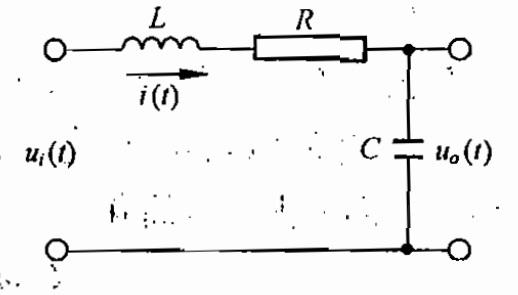其输入输出关系的微分方程为：
$LC \frac {d^2 u_o(t)}{dt^2} + RC \frac {d u_o(t)}{dt} +u_o(t) =u_i(t)$
对上式进行拉普拉斯变换得到:
$L[ \frac {d u_o(t)}{dt} ] = sU_o(s) -u_o(0)$
$L[ \frac {d^2 u_0(t)}{dt^2} ] = s^2U_o(s) -s u_o(0)-u_o^{'}(0)$
整理可得：
$LC (s^2U_o(s) -s u_o(0)-u_o^{'}(0)) +RC(sU_o(s) -u_o(0))+ U_o(s)=U_i(s)$


展开全文• FUN = INVLAPFUN(B,A) 返回个函数句柄，用于评估与拉普拉斯变换 B(s)/A(s) 关联的时间函数 FUN(t)，其中 B 和 A 是包含多项式系数的相应行向量。 FUN = INVLAPFUN(TF) 使用 Control Toolbox 传递函数对象 TF。 ...matlab
• 但是，一般的教材上来就是拉普拉斯变换的数学定义，对于其历史和代表的深刻含义没有任何介绍，导致很多人一直头疼不已。今天，我们尝试一下从不同的角度来看看拉普拉斯变换到底是怎么回事？先从个人说起：奥列弗...
• FUN = INVLAPFUN(B,A) 返回个函数句柄，用于评估与拉普拉斯变换 B(s)/A(s) 关联的时间函数 FUN(t)，其中 B 和 A 是包含多项式系数的相应行向量。 FUN = INVLAPFUN(TF) 使用 Control Toolbox 传递函数对象 TF。 ...matlab
• 拉普拉斯变换种数学积分变换，其核心是把时间函数f(t)与复变函数F(s)联系起来，把时域问题通过数学变换为复频域问题，把时域的高阶微分方程变换为频域的代数方程以便求解。 拉普拉斯变换学习笔记 ...
• ## 拉普拉斯变换详解

千次阅读 2018-11-07 22:01:26
拉普拉斯变换的定义 ...拉普拉斯变换种数学积分变换，其核心是把时间函数f(t)与复变函数F(s)联系起来，把时域问题通过数学变换为复频域问题，把时域的高阶微分方程变换为频域的代数方程以便求解。  ...
• 2、拉普拉斯变换是工程数学中常用的种积分变换，又名拉氏转换，其符号为。拉氏变换是个线性变换，可将个有引数实数t（t ≥ 0）的函数转换为个引数为复数s的函数。有些情形下个实变量函数在实数域中进行...
• ## 拉普拉斯变换学习笔记

千次阅读 多人点赞 2019-05-06 09:40:44
1.为什么引入拉普拉斯变换？ 2.双边拉普拉斯的定义 3.双边拉普拉斯变换的收敛域 4.单边拉普拉斯变换的定义 5.单边拉普拉斯变换和傅立叶变换的关系 6.常见信号的拉式变换 7.拉普拉斯变换的性质 7.1.线性、...
• 精品文档 实验六 拉普拉斯变换及其逆变换 目的 1掌握连续系统及信号拉普拉斯变换概念 2 掌握利用 MATLAB绘制系统零极点图的方法 3 掌握利用 MATLAB求解拉普拉斯逆变换的方法 二拉普拉斯变换曲面图的绘制 连续时间...
• 给出了运用拉普拉斯变换求解常系数线性自治动力系统满足在任意点的初值条件的解及其通解的方法。
• 学生角度看傅里叶变换，拉普拉斯变换，z变换（） 学生角度看傅里叶变换，拉普拉斯变换，z变换（） 离散和连续信号的表示 信号的脉冲表示 响应与卷积 傅里叶变换 为什么需要傅里叶变换 ejwtejwte^{jwt}的...
• ## OpenCV拉普拉斯变换

千次阅读 2016-04-26 17:08:52
拉帕拉斯变换用来检测物体的边缘信息。 在图像平坦（灰度值无变换的区域），拉普拉斯滤波后的图像在该区域的强度值为0.在图像灰度值剧烈变换的区域（边缘），拉普拉斯滤波后的...拉普拉斯变换变换可用于图像增强opencv 图像增强
• 本文主要讲了一下关于拉普拉斯变换的应用在电路设计，起来学习一下
• 拉普拉斯变换定义 要知道不是所有函数傅里叶变换都存在，为了找到收敛变换，在傅里叶变换基础上，信号乘以个衰减因子e−σte^{-\sigma t}e−σt于是得到拉普拉斯变换对： F(s)=∫0∞f(t)e−stdtf(t)=12π∫σ...信号处理...